# High School Physics : Types of Waves

## Example Questions

### Example Question #969 : High School Physics

In seismology, the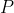wave is a longitudinal wave.  As anwave travels through the Earth, the relative motion between thewave and the particles is

Perpendicular

First perpendicular, then parallel

First parallel, then perpendicular

Parallel

Parallel

Explanation:

Longitudinal waves are waves whose particles travel parallel to the direction that the wave itself is traveling.  Sound waves are another example of longitudinal waves.

### Example Question #1 : Types Of Waves

Which of the following waves require a medium to travel?

Sound Waves

Light Waves

Microwaves

Sound Waves

Explanation:

Electromagnetic waves are the only  type of wave that do not require a medium to travel.  Light, radio and microwaves are examples of electromagnetic waves.  Sound does require a medium to travel.  In a vacuum sound waves cannot travel as there is no air to compress.

### Example Question #971 : High School Physics

In a vacuum, the velocity of all electromagnetic waves

depends on the amplitude

Is zero

depends on the frequency

Is nearly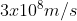Is nearlyExplanation:

Electromagnetic waves all travel at the same speed which is the speed of light.  The speed of light in a vacuum is nearly### Example Question #46 : Waves

A radio station broadcasts at a frequency of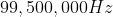. If the broadcast is an electromagnetic wave, then what is its wavelength?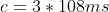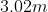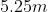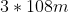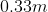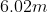Explanation:

The relationship between wavelength and frequency is given by the equation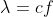, where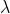is the wavelength,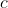is the speed of light, and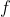is frequency.

We are given the values for frequency and the speed of light, allowing us to solve for the wavelength.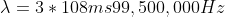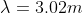### Example Question #47 : Waves

In seismology, the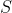wave is a transverse wave.  As anwave travels through the Earth, the relative motion between thewave and the particles is

First perpendicular, then parallel

Parallel

First parallel, then perpendicular

Perpendicular